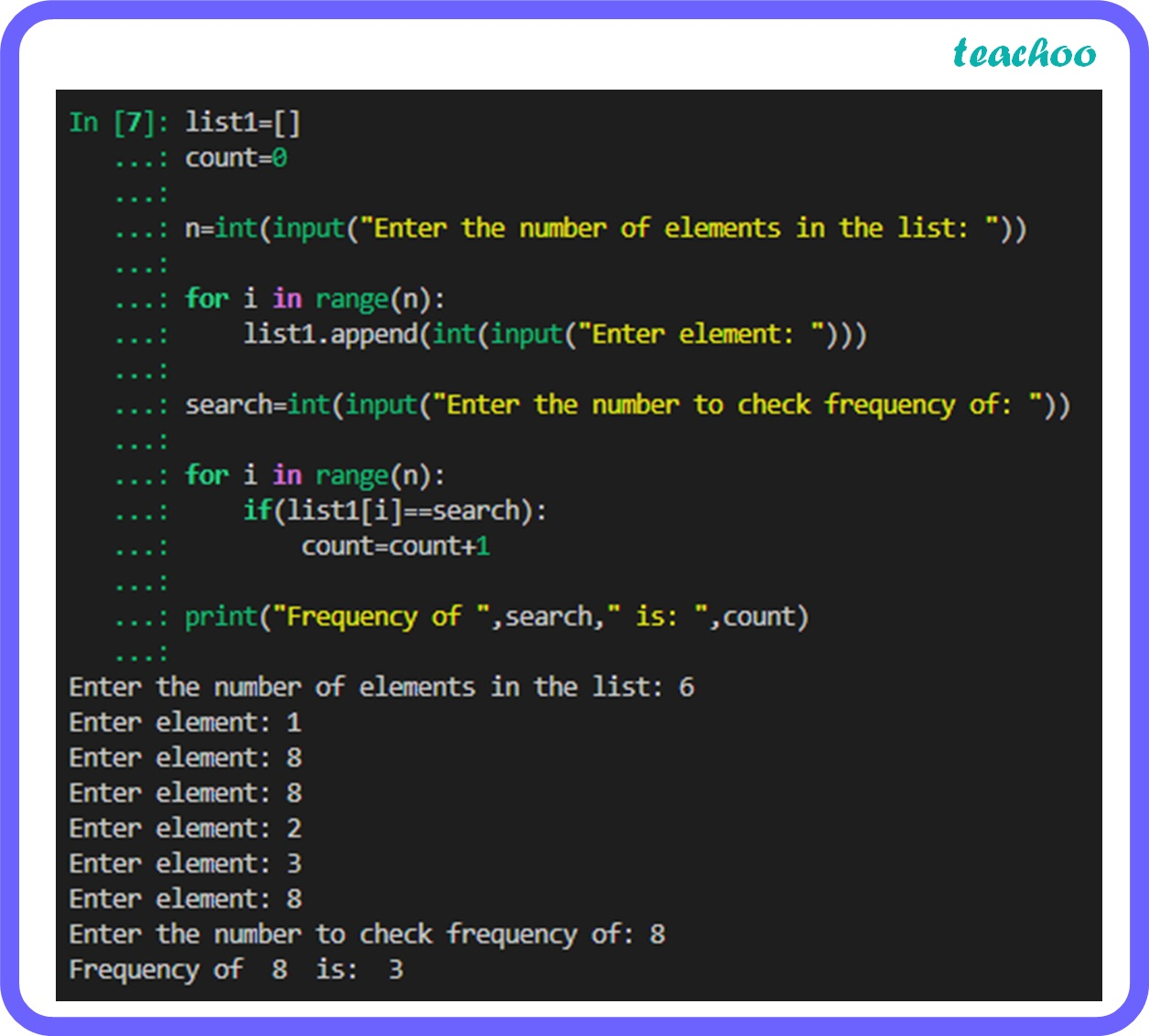Long Answer Type Questions (5 Marks each)

Computer Science - Class 11
Chapter 9 Class 11 - Lists

## Write a python program to find the frequency of a number in a list.

Code:

``` list1=[]  ```

``` count= 0 ```

``` n=int(input( "Enter the number of elements in the list: " )) ```

``` for i in range(n): ```

```     list1.append(int(input( "Enter element: " ))) ```

``` search=int(input( "Enter the number to check frequency of: " )) ```

``` for i in range(n): ```

```     if(list1[i]==search): ```

```         count=count+ 1 ```

``` print("Frequency of ",search," is: ",count) ```Learn in your speed, with individual attention - Teachoo Maths 1-on-1 Class